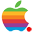# Microsoft Excel 中的函数与公式：有什么区别？

1年前 (2021-12-03) 671次浏览

## Excel中的函数是什么？

• 数数
• 平均数
• 中转
• 修剪

Excel 中的每个函数都需要特定的语法或组合。当您插入一个函数来帮助您创建公式时，您可以看到此语法。例如，如果单击编辑栏左侧的“插入函数”图标 (fx)，则可以搜索要插入的函数。

## Excel中的公式是什么？

Excel 中的公式是您在单元格内创建的表达式，例如方程。您可以在公式中插入一个函数或创建一个没有函数的方程。

### 带函数的公式

•  `=SUM(A1:A10)`
•  `=COUNT(A1:A10)`
•  `=AVERAGE(A1:A10)`
•  `=TRUNC(7.5,1)`
•  `=TRIM(A1)`

### 没有函数的公式

•  `=A1+A2`
•  `=C1-C2`
•  `=2*4`
•  `=B1/B2`
•  `=D1*D2`

## 记住函数和公式之间的区别

• 功能：Excel预定义，可插入公式。
• 公式：由您定义，可以与函数一起使用，也可以不与函数一起使用。

TobMAC , 版权所有丨如未注明 , 均为原创丨本网站采用BY-NC-SA协议进行授权